What does it do?

Creates an array of sequential numbers

Formula breakdown:

=SEQUENCE(rows, [columns], [start], [step])

What it means:

=SEQUENCE(number of rows, [number of columns], [starting number], [increment per number])

Did you know that you can now generate a series of numbers with an Excel Formula? Yes you can! It is definitely possible now with Excel’s SEQUENCE Formula. It is a new formula introduced in Office 365 released in 2018!

I explain how you can do this below:STEP 1: We need to enter the SEQUENCE function in a blank cell:

## =SEQUENCE(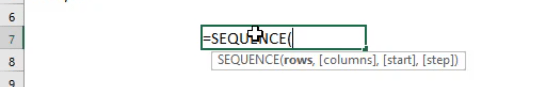STEP 2: The SEQUENCE arguments:

## rows

How many rows to fill with values?

Let us go for 10 rows.

## =SEQUENCE(10,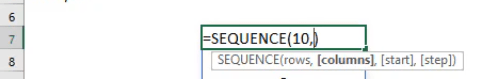## [columns]

How many columns to fill with values?

Let us go for 3 columns.

## =SEQUENCE(10, 3,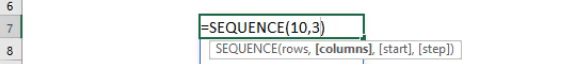## [start]

Which number do you want the sequence of numbers to start?

Let us have it start at the number 10.

## =SEQUENCE(10, 3, 10,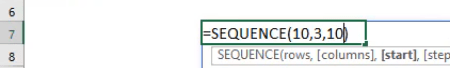## [step]

Which increment for each number in the sequence?

Let us increment it by 100. So that numbers should look like: 10, 110, 210, 310, and so on…

## =SEQUENCE(10, 3, 10, 100)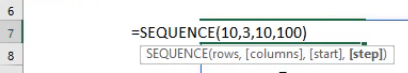Try it out now and see that your sequence of numbers is generated magically!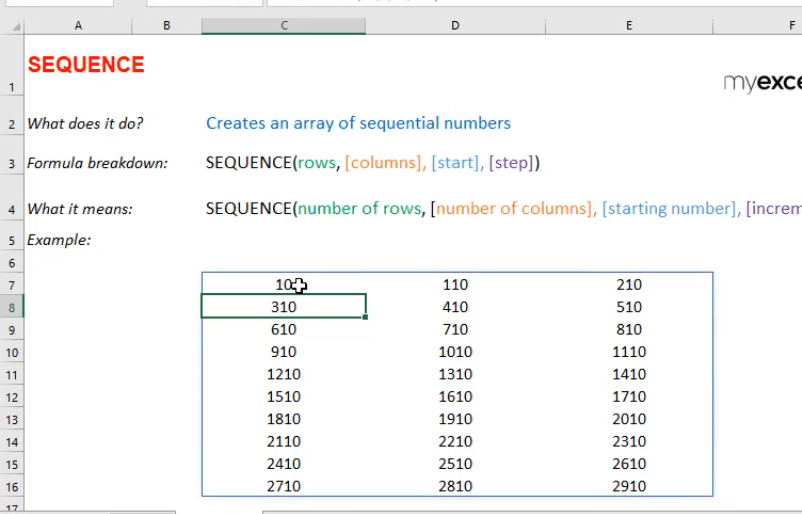How to Use the SEQUENCE Formula in Excel